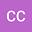Discrete Lyapunov function by means of a discrete residual energy function and stability analysis for discrete time physical systems
•## Abstract

In this research paper beginning from the fundamental concepts, all the basic approach is introduced for short. Then after obtaining the discrete Lagrange-dissipative model ({L,D}- model for short) of a discrete time observed physical/engineering dynamic system, the model will be used to develope the discrete Hamiltonian together with discrete dissipation energy as discrete Lyapunov function and as such, a systematic method is proposed to obtain discrete Lyapunov function in form of a residual energy function for discrete (time) observed physical systems to analyze the discrete time system related to stability. Stability analysis of a discrete time observed physical dynamic system is performed using discrete Lyapunov function in form of a residual energy function consisting of Hamiltonian together with dissipation energy. Application of the method was shown using two discrete time physical examples, one of which is a coupled one and time dependent. This coupled physical discrete time example is analyzed related to stability using two different formulations, one form of which leads to a result.

#### Peer review status:UNDER REVIEW

24 Jul 2021Submitted to Mathematical Methods in the Applied Sciences
26 Jul 2021Assigned to Editor
26 Jul 2021Submission Checks Completed
01 Aug 2021Reviewer(s) Assigned